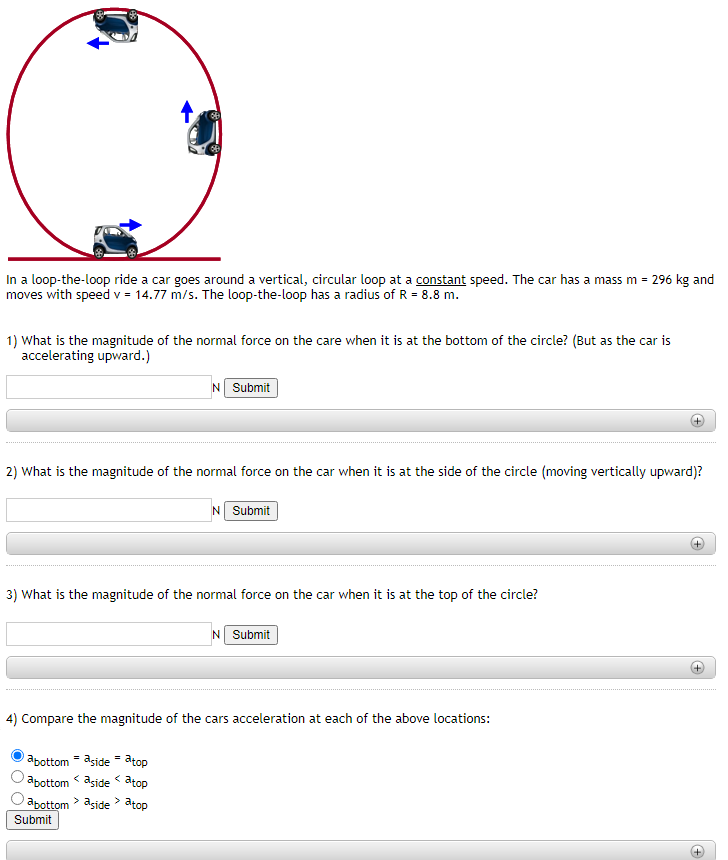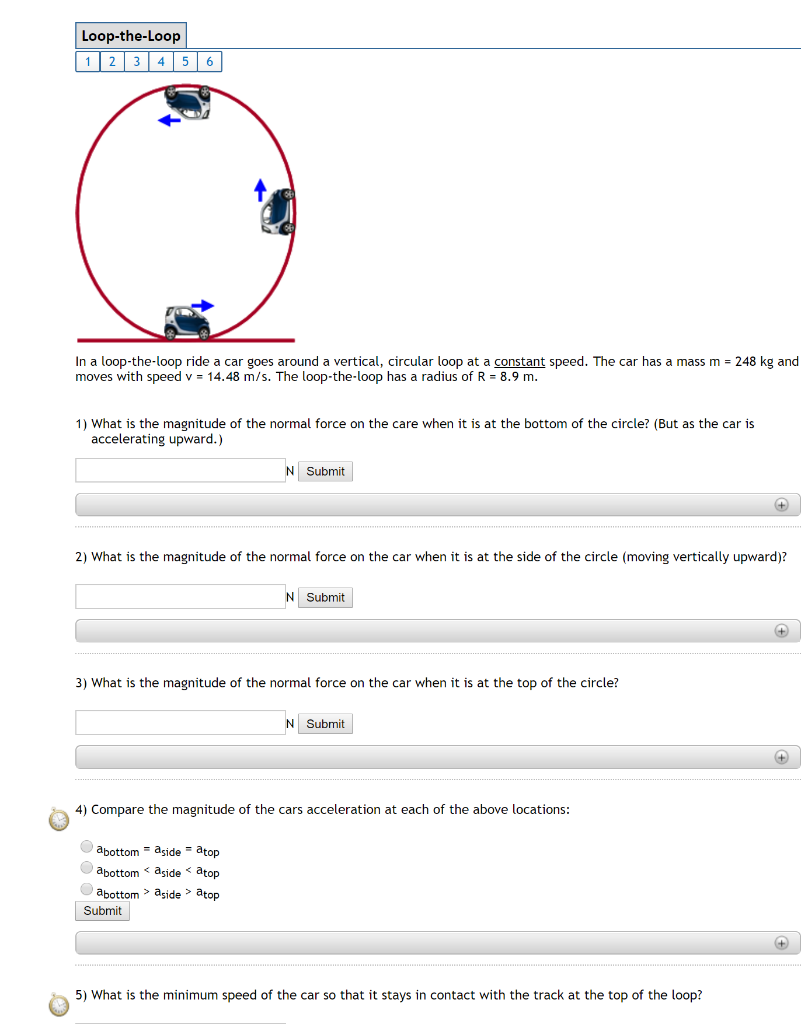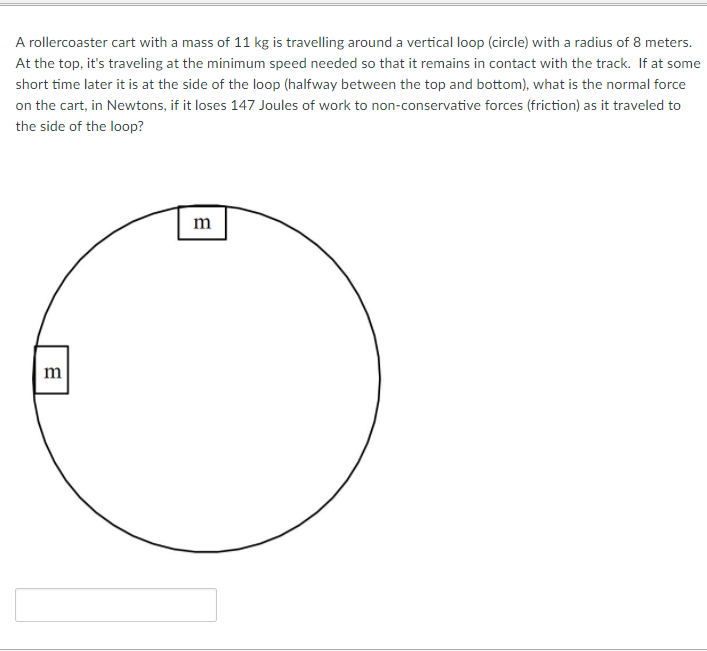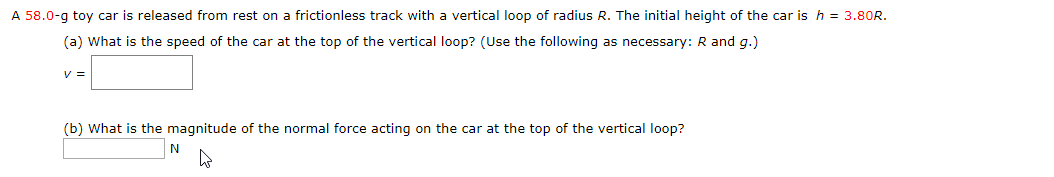Question

# In a loop-the-loop ride a car goes around a vertical, circular loop at a constant speed....

In a loop-the-loop ride a car goes around a vertical, circular loop at a constant speed. The car has a mass m = 286 kg and moves with speed v = 13.82 m/s. The loop-the-loop has a radius of R = 8 m.

1)

What is the magnitude of the normal force on the care when it is at the bottom of the circle? (But as the car is accelerating upward.)

2)

What is the magnitude of the normal force on the car when it is at the side of the circle (moving vertically upward)?

3)

What is the magnitude of the normal force on the car when it is at the top of the circle?

4)

Compare the magnitude of the cars acceleration at each of the above locations:

A) abottom = aside = atop

B) abottom < aside < atop

C) abottom > aside > atop

5)

What is the minimum speed of the car so that it stays in contact with the track at the top of the loop?

Given, m = 286 kg

v = 13.82 m/s

R = 8 m

Centripetal force, Fc = mv^2 /R = 286*(13.82)^2 /8 = 6827.98 N

1) At the bottom of the circle,

F = Fc + mg = 6827.98 + 280*9.81 = 9574.78 N

2) At the side of the circle,

F = Fc = 6827.98 N

3) At the top of the circle,

F = Fc - mg = 6827.98 - 280*9.81 = 4081.18 N

4) a(bottom) = 9574.78/280 = 34.2 m/s^2

a(side) = 6827.98/280 = 24.38 m/s^2

a(top) = 4081.18/280 = 14.57 m/s^2

Option C is correct.

a(bottom) > a(side) > a(top)

5) Minimum speed = sqrt(g*r) = sqrt(9.81*8) = 8.86 m/s

#### Earn Coins

Coins can be redeemed for fabulous gifts.

Similar Homework Help Questions
• ### In a loop-the-loop ride a car goes around a vertical, circular loop at a constant speed....In a loop-the-loop ride a car goes around a vertical, circular loop at a constant speed. The car has a mass m = 296 kg and moves with speed v = 14.77 m/s. The loop-the-loop has a radius of R = 8.8 m. 1) What is the magnitude of the normal force on the care when it is at the bottom of the circle? (But as the car is accelerating upward.) N Submit 2) What is the magnitude of the...

• ### 1 Loop-the-Loop 1 23 45 6 In a loop the loop ride a car goes around...1 Loop-the-Loop 1 23 45 6 In a loop the loop ride a car goes around a vertical circular loop at a constant speed. The car has a mass m = 248 kg and moves with speed v- 14.48 m/s. The loop-the-loop has a radius of R -8.9 m. 1) What is the magnitude of the normal force on the care when it is at the bottom of the circle? (But as the car is accelerating upward.) Nnii 2) What...

• ### A rollercoaster cart with a mass of 11 kg is travelling around a vertical loop (circle)...A rollercoaster cart with a mass of 11 kg is travelling around a vertical loop (circle) with a radius of 8 meters. At the top, it's traveling at the minimum speed needed so that it remains in contact with the track. If at some short time later it is at the side of the loop (halfway between the top and bottom), what is the normal force on the cart, in Newtons, if it loses 147 Joules of work to non-conservative...

• ### A car in an amusement park roller coaster ride rolls without friction at the top of...

A car in an amusement park roller coaster ride rolls without friction at the top of a hill. The car begins at a height h from the top of a hill. A the bottom, the car then goes through a vertical loop where the car is upside down at the loop\'s top. If the radius of the loop is 20.0m, what is the minimum height h such that the car moves around the loop without falling off the track at...

• ### A car travels at a constant speed around a circular track whose radius is 2.65 km. The car goes once around the track i...

A car travels at a constant speed around a circular track whose radius is 2.65 km. The car goes once around the track in 341 s. What is the magnitude of the centripetal acceleration of the car?

• ### A car goes around an icy banked curve (frictionless) at a speed that is not too...

A car goes around an icy banked curve (frictionless) at a speed that is not too fast so that the car stays on the circula path. What causes the car to follow the circular path? A car goes around an icy banked curve (frictionless) at a speed that is not too fast so that the car stays on the circula path. What causes the car to follow the circular path? the normal force from the road gravity the friction force...

• ### A small car with mass 0.670 kg travels at constant speed, find the normal force!

A small car with mass 0.670 kg travels at constant speed on the inside of a track that is a vertical circle with radius 5.00 m the following figure.If the normal force exerted by the track on the car when it is at the top of the track (point B) is 6.00 N, what is the normal force on the car when it is at the bottom of the track (point A)?

• ### A 48.5-g toy car is released from rest on a frictionless track with a vertical loop...

A 48.5-g toy car is released from rest on a frictionless track with a vertical loop of radius R. The initial height of the car is h = 4.45R. (a) What is the speed of the car at the top of the vertical loop? (Use the following as necessary: R and g.) (b) What is the magnitude of the normal force acting on the car at the top of the vertical loop?

• ### A 58.0-g toy car is released from rest on a frictionless track with a vertical loop...A 58.0-g toy car is released from rest on a frictionless track with a vertical loop of radius R. The initial height of the car is h 3.80R. (a) What is the speed of the car at the top of the vertical loop? (Use the following as necessary: R and g.) v= (b) What is the magnitude of the normal force acting on the car at the top of the vertical loop?

• ### A small remote-controlled car with mass 1.60 kg moves at a constant speed of v =...

A small remote-controlled car with mass 1.60 kg moves at a constant speed of v = 12.0 m/s in a track formed by a vertical circle inside a hollow metal cylinder that has a radius of 5.00 m, see Fig below. What is the magnitude of the normal force exerted on the car by the walls of the cylinder at a) point A (bottom of the track)? b) point B (top of the track)?